# Basic functions - math word problems

#### Number of problems found: 2468

• Age ratioJanko is 14 years old. The age ratio of Janka and Zuzka is 2: 3. What was the ratio seven years ago?
• Rate 3A liquid is heated at a rate of 5 degrees Celsius per minute. If the temperature now is at 39 degrees Celsius, how long will the temperature by 94 degrees Celsius?
• Water current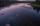John swims upstream. After a while, he passes the bottle, from that moment he floats for 20 minutes in the same direction. He then turns around and swims back, and from the first meeting with the bottle, he sails 2 kilometers before he reaches the bottle.
• Storm and roof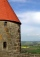The roof on the building is a cone with a height of 3 meters and a radius equal to half the height of the roof. How many m2 of roof need to be repaired if 20% were damaged in a storm?
• The bus stop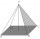The bus stop waiting room has the shape of a regular quadrilateral pyramid 4 m high with a 5 m base edge. Calculate how much m2 roofing is required to cover the sheathing three walls, taking 40% of the additional coverage.
• NitrogenOne bag of urea containing 46 percent nitrogen weighs 25 kg. How many bags have to be purchased for fertilizing a field of 41003 square meters if the nitrogen dose is 50.0 kg per hectare?
• DivideHow many different ways can three people divide 7 pears and 5 apples?
• Two accounts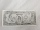Two accounts in the bank, one per year interest 2%, the second 3%. Total interest income 1900 USD. If interest rates were reversed, the yield would be USD 200 higher. What are the amounts on each account?
• The fastestThe fastest boat can reach speeds more than 710% as fast as the Queen Mary 2. How would you express this number as a fraction and as a decimal?
• The ballThe ball has a radius of 2m. What percentage of the surface and volume is another sphere whose radius is 20% larger?
• Same force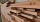The trunk of 5m length and 95 kilograms has a center of gravity 2m from the thicker end. The tribe is carried by two men, one at the thicker end. At what distance does the trunk carry a man from the other end to make the same force on it?
• TV competitionIn the competition, 10 contestants answer five questions, one question per round. Anyone who answers correctly will receive as many points as the number of competitors answered incorrectly in that round. After the contest, one of the contestants said: We
• FruitsIn the shop sell 4 kinds of fruits. How many ways can we buy three pieces of fruit?
• Bouquets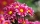In the flower shop they sell roses, tulips and daffodils. How many different bouquets of 5 flowers can we made?
• Twelve flowersA florist has roses, tulips, daffodils, and carnations to use in flower arrangements. If she were to make an arrangement using 12 flowers, how many different combinations of these 4 types of flowers would be possible?
• Possible combinations - word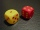How many ways can the letters F, A, I, R be arranged?
• Conical bottleWhen a conical bottle rests on its flat base, the water in the bottle is 8 cm from it vertex. When the same conical bottle is turned upside down, the water level is 2 cm from its base. What is the height of the bottle?
• Two bodiesThe rectangle with dimensions 8 cm and 4 cm is rotated 360º first around the longer side to form the first body. Then, we similarly rotate the rectangle around the shorter side b to form a second body. Determine the ratio of surfaces of the first and seco
• Pizza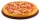Three siblings ordered one pizza. Miška ate a quarter of the whole pizza. Lenka ate a third of the rest and Patrik ate half of what Lenka had left. They had the rest packed up. How much of the pizza did they pack? Write the result as a fraction.
• Diagonal intersectisosceles trapezoid ABCD with length bases | AB | = 6 cm, CD | = 4 cm is divided into 4 triangles by the diagonals intersecting at point S. How much of the area of the trapezoid are ABS and CDS triangles?

Do you have an interesting mathematical word problem that you can't solve it? Submit a math problem, and we can try to solve it.

We will send a solution to your e-mail address. Solved examples are also published here. Please enter the e-mail correctly and check whether you don't have a full mailbox.

Please do not submit problems from current active competitions such as Mathematical Olympiad, correspondence seminars etc...